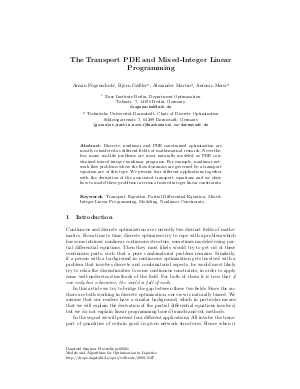Document# The Transport PDE and Mixed-Integer Linear Programming

### Authors Armin Fügenschuh, Björn Geißler, Alexander Martin, Antonio Morsi## File

DagSemProc.09261.31.pdf
• Filesize: 164 kB
• 9 pages

## Cite As

Armin Fügenschuh, Björn Geißler, Alexander Martin, and Antonio Morsi. The Transport PDE and Mixed-Integer Linear Programming. In Models and Algorithms for Optimization in Logistics. Dagstuhl Seminar Proceedings, Volume 9261, pp. 1-9, Schloss Dagstuhl - Leibniz-Zentrum für Informatik (2009)
https://doi.org/10.4230/DagSemProc.09261.31

## Abstract

Discrete, nonlinear and PDE constrained optimization are mostly considered as different fields of mathematical research. Nevertheless many real-life problems are most naturally modeled as PDE constrained mixed integer nonlinear programs. For example, nonlinear network flow problems where the flow dynamics are governed by a transport equation are of this type. We present four different applications together with the derivation of the associated transport equations and we show how to model these problems in terms of mixed integer linear constraints.
##### Keywords
• Transport Equation
• Partial Differential Equation
• Mixed-Integer Linear Programming
• Modeling
• Nonlinear Constraints

## Metrics

• Access Statistics
• Total Accesses (updated on a weekly basis)
0